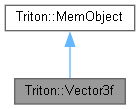Triton Visual simulation library for ocean rendering.
Triton::Vector3f Class Reference

A 3D single-precision vector class, and its operations. More...

`#include <Vector3.h>`

Inheritance diagram for Triton::Vector3f:[legend]
Collaboration diagram for Triton::Vector3f:[legend]

## Public Member Functions

Vector3f ()
Default constructor; does not initialize the vector. More...

Vector3f (const Triton::Vector3 &v)
Construct a single precision vector from a double precision one. More...

Vector3f (float px, float py, float pz)
Constructs a Vector3f from the specified single-precision floating point x, y, and z values. More...

float TRITONAPI Length ()
Returns the length of this vector. More...

void TRITONAPI Normalize ()
Scales the vector to be of length 1.0. More...

double TRITONAPI Dot (const Vector3f &v) const
Returns the dot product of this vector with the specified Vector3. More...

Vector3f TRITONAPI operator - (const Vector3f &v) const
Subtracts the specified vector from this vector, and returns the result. More...

Vector3f TRITONAPI operator+ (const Vector3f &v) const
Adds this vector to the specified vector, and returns the result. More...

Vector3f TRITONAPI operator * (const Vector3f &v) const
Multiplies the components of two vectors together, and returns the result. More...

Vector3f TRITONAPI operator * (float n) const
Scales each x,y,z value of the vector by a constant n, and returns the result. More...

## Public Attributes

float x
Data members x, y, z are public for convenience. More...

## Detailed Description

A 3D single-precision vector class, and its operations.

## ◆ Vector3f() [1/3]

 Triton::Vector3f::Vector3f ( )
inline

Default constructor; does not initialize the vector.

## ◆ Vector3f() [2/3]

 Triton::Vector3f::Vector3f ( const Triton::Vector3 & v )
inline

Construct a single precision vector from a double precision one.

## ◆ Vector3f() [3/3]

 Triton::Vector3f::Vector3f ( float px, float py, float pz )
inline

Constructs a Vector3f from the specified single-precision floating point x, y, and z values.

## ◆ Dot()

 double TRITONAPI Triton::Vector3f::Dot ( const Vector3f & v ) const
inline

Returns the dot product of this vector with the specified Vector3.

## ◆ Length()

 float TRITONAPI Triton::Vector3f::Length ( )
inline

Returns the length of this vector.

## ◆ Normalize()

 void TRITONAPI Triton::Vector3f::Normalize ( )
inline

Scales the vector to be of length 1.0.

## ◆ operator *() [1/2]

 Vector3f TRITONAPI Triton::Vector3f::operator * ( const Vector3f & v ) const
inline

Multiplies the components of two vectors together, and returns the result.

## ◆ operator *() [2/2]

 Vector3f TRITONAPI Triton::Vector3f::operator * ( float n ) const
inline

Scales each x,y,z value of the vector by a constant n, and returns the result.

## ◆ operator -()

 Vector3f TRITONAPI Triton::Vector3f::operator - ( const Vector3f & v ) const
inline

Subtracts the specified vector from this vector, and returns the result.

## ◆ operator+()

 Vector3f TRITONAPI Triton::Vector3f::operator+ ( const Vector3f & v ) const
inline

Adds this vector to the specified vector, and returns the result.

## ◆ x

 float Triton::Vector3f::x

Data members x, y, z are public for convenience.

The documentation for this class was generated from the following file: